\$B:#>,\$N0lGU(B

\$BKhF|!":#>,\$N0lGU\$r>e\$2\$F\$\$\$^\$9!#(B
\$B:#>,0{\$`\$b\$N\$K:\$\$C\$?\$i!";29M\$K\$7\$F\$_\$F\$/\$@\$5\$\$!#(B

2020\$BG/(B8\$B7n(B

 1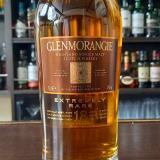(I8^Z]S0Z]<^(B18\$BG/(B 2 \$B5Y\$_(B 3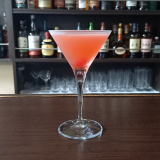(I?Y%C^%M^W0I(B 4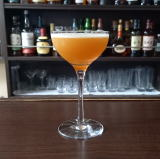(I<^,O26%JF0K^0(B 5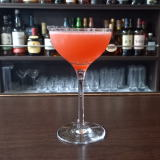(I=D[M^X0%L(0Y=^(B 6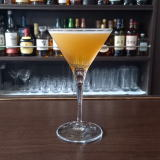(ID0K_0D^(B 7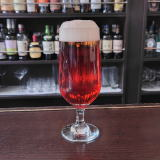(I6]J_X%K^1(B 8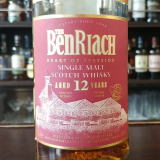(IM^]X1/8(B12\$BG/(I<*X0(B 9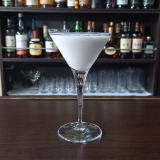(IT]70%D^)0D^Y(B 10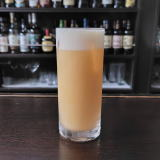(I@^0K^0%L(=^(B 11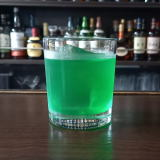(I:^<^W(B 12(I\27X(B 13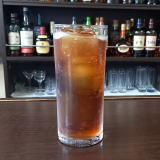(I6XK^1]%:0W(B 14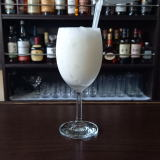(I::E/B%D^X0Q(B 15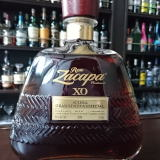(I[]%;6J_(BXO 16 \$B5Y\$_(B 17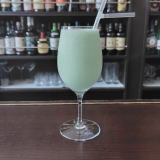(IL^Y0%J\21](B 18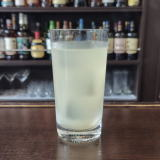(IS]70%Z]A(B 19(IL^W/<]8^%3YL(B 20(IK_10=D%8X(B 21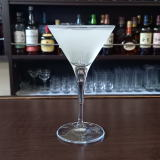(I1/L_YC(0F(B 22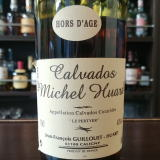(IP/<*Y%U10Y(B 23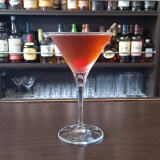(IO]J/@]\$B!!(IPC^(1Q(B 24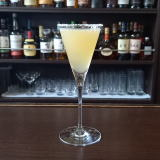(I8^W]OYF4%OY6^X0@(B 25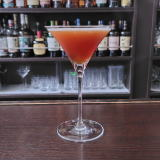(IZ2%[]8^(B 26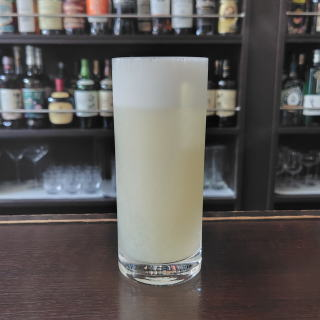(I 27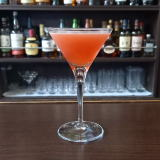(IK_0@0%@\0(B 28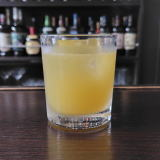(IS]70%P/8=(B 29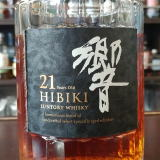\$B6A(B21\$BG/(B 30 \$B5Y\$_(B 31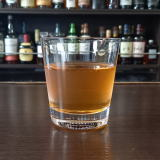(I;>^W/8(B